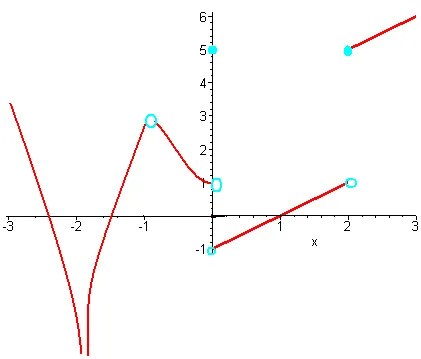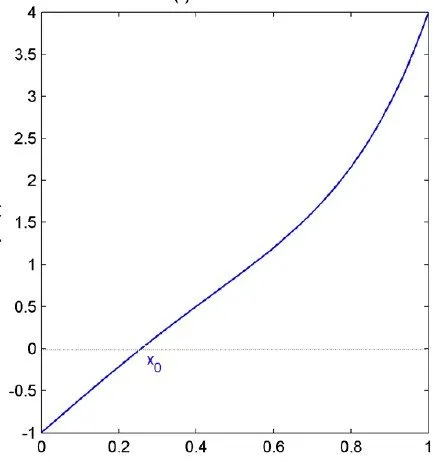# Mathematics - Mathematical Analysis Continuity IIHello its me again drifter1! Today we continue with Mathematical Analysis talking a little more about Continuity and getting also into some examples! You should check out at least my previous post so that you have a better understanding, because I will continue getting into more specific things about what we already talked about...So, let's get started!

## Bolzano Theorem:

Continuity can be useful in many things that have to do with functions. One of these is finding if a function has a solution in a specific range (a, b). The Bolzano Theorem is used to find out if a function f: [a, b] -> R that is continuous in that range has a solution f(x0) = 0, where a < x0 < b

When f(a)*f(b) < 0 then there is atleast one x0 in (a, b) so that f(x0) = 0

So, using this theorem we can find out if a solution exists in that range. But, we can't find out if there are more. To find out if there are more we would have to split the range (a, b) into subranges and check if the Bolzano Theorem applies to more than 1 of them. That way we would also find a approximately value for that solution.

Example:

Suppose the polynomial function f(x) = 3x^5 - 2x^3 + 4x - 1.

Is there a solution in the range (0, 1)?

f(0) = -1, f(1) = 4 and so f(0) * f(1) = -4 < 0

Using the Bolzano theorem we know that there is atleast one solution in that range.

If we make the range smaller let's say (0.2, 0.3) we will see that the Bolzano theorem applies again and that means that the solution is in between of 0.2 and 0.3.

Plotting the graph of the function we see that the solution f(x0) = 0 is really in that range!## Intermediate Value Theorem:

Suppose a function f(x) : [a, b] -> R again, that is continuous in that range and where f(a) != f(b). For every value c in between of f(a) and f(b) we know that there is a number x0 in the range (a, b) so that f(x0) = c.

Try proving it using the Bolzano theorem :)

To understand this theorem you have to think about the geometric representation using the graph of a continuous function. Look at the graph of the polynomial function we had in our Example previously. You can see that when going in any range (a, b) the function will contain all the "images" f(x) that are in between of f(a) and f(b). For example in (0, 1) we have f(0) = -1 and f(4) = 4 and so there is a x0 in f so that f(x0) is equal to any value in between of -1 and 4. This means that if that value is 0 we know that there is a solution in that range in the same way as we proved it using the Bolzano theorem!

## Continuity and Boundness:

Suppose a function f that is continuous in the range [a, b].

Then we also know that:

• f is also bounded in [a, b]
• there are real number x1, x2 so that f(x1) = minf = m and f(x2) = maxf = M
• f([a, b]) = [m, M] which means that the images of f are in the range [m, M]

## Continuity and Composition:

Suppose the function f: A->R and g: B->R where f(A) is a subset of B. When f is continuous in the point x0 and g is continuous in the point f(x0) then the composition of f and g, gof, is also continuous in x0. We can generallize this by saying that if f is continous in the set A and g is continuous in the set f(A) then the composition gof is also continuous in A.

## Continuous Function Examples:

We can now say that if f(x) is continous in R the following are also continuous as compositions of continous functions.

• Trigonometric functions sin(f(x)), cos(f(x)), tan(f(x)) and cot(f(x))
• Hyperbolic functions sinh(f(x)), cosh(f(x)), tanh(f(x)) and coth(f(x))
• Exponential functions e^f(x), a^f(x) where a > 0
• Logarithmic functions loga(f(x)) where a, f(x) > 0
• Root functions root(f(x)) where f(x) >= 0

Inverse Functions:

If the function f(x): A->R is 1-1 and continuous we know that the inverse function f^-1: f(A)->A is also continuous. We get the same result when function f is strictly monotonic (increasing or decreasing) and continuous. This means that the following functions are also continuous:

• Inverse Trigonometric Functions arcsin(f(x)), arccos(f(x)), arctan(f(x)) and  arccot(f(x))
• Inverse Hyperbolic Functions arcsinh(f(x)), arccosh(f(x)), arctanh(f(x)) and arccoth(f(x))

So, this means that the logarithmic and exponential functions can be proven continuous by proving that either one is continuous, because the one is the inverse of the other.

Examples:

1. Is the function ln(x^2 + 1) continuous?

Well, the function is a composition (gof) of g(x) = lnx and f(x) = x^2 +1.

This means that is is continuous as the result of a composition of two continuous functions.

2. In which range is the function ln(1 - x^2) continuous?

The function is a result of the composition of f(x) = 1 - x^2 and g(x) = lnx.

But, because f(x) can get negative values and x in lnx must be positive we need to find out when f(x) is > 0.

f(x) = 1 - x^2 >= 0

x^2 <= 1 -> x > -1 and x < 1.

So, the compositive function is continuous only in the range (-1, 1), where x^2 is less then 1 and so the result is (strictly) positive and non-zero.

## Continuity proof Examples:

Let's now finally get into some examples where we will check if the functions are continuous or not!

1. Suppose f(x) = x^2 + 1, when x <0

= 2, when x == 0

= 2x + 1, when x > 0

Is the function continuous?

We know that each sub-function is continuous, because they are polynomial or constant function, but what about the point x = 0?

We already see that f(0) = 2 so let's find out if the one-sided limits for 0 are also equal to 2.

lim x->0+ (f(x)) = lim x->0+ (2x + 1) = 2*0 + 1 = 1 (already proven that we have a discontinuity)

lim x->0- (f(x)) = lim x->0- (x^2 + 1) = 0 + 1 = 1

The two one-sided limits are equal, but f(x) is not equal to 1 in that point, but 2 and so the function f is discontinuous at the point x = 0.

2. Suppose the function g(x) = x + 1, when x <= 1

= 3 - ax^2, when x > 1

For which values of a the function g is continuous?

Well, both are continuous again as polynomial functions and so we will have to find the value of a so that the limit to 1 of the second part is equal to x + 1 = 1 + 1 = 2.

lim x->1+ g(x) = 2 => lim x->1+ (3 - ax^2) = 2 => 3 - a = 2 => a = 1.

So, the function is continuous only when a = 1 that means that we don't have a discontinuity at the point x = 1, because we already know that the remaining part is continuous anyway.

If we also had a one-sided limit from the left we would create a linear system and solve it so that we find the value of a (or even more parameters).

And this is actually it and I hope you liked it!

Next time we will get into Derivatives!

Until next time...Bye!

H2
H3
H4
3 columns
2 columns
1 column